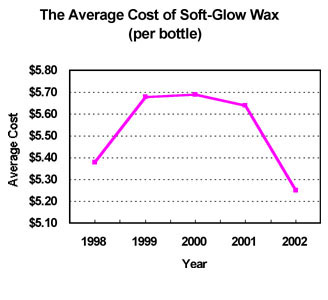# Approximately how much did the cost of Soft-Glow Wax increase from 1998 to 1999?$$\0.30$$

Explanation

In 1998, the cost of Soft-Glow Wax was approximately $$\5.38$$. In 1999, the cost was approximately $$\5.68$$. Subtract to find the increase.